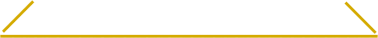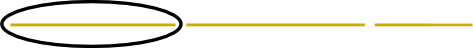Here’s a fun little math puzzle:

Suppose you have a spaghetti straw that you randomly break into three pieces. What’s the probability that you can form a triangle out of those three pieces?

Here’s a solution that I and Frej Berglind came up with.

First we note that it’s not always possible to form a triangle. Take this case for example:Looking at the picture above, one quickly realizes that the two shortest pieces must be at least as long as the longest piece for it to be possible to form a triangle. This gives us a different formulation of the problem:

What’s the probability that none of the pieces are longer than half of the spaghetti straw?

Now, it’s slightly easier if we turn the question around: What is the probability of one piece being longer than half the spaghetti straw?

We start by focusing on the left most piece:What’s the probability that this piece ends up being more than half of the full length? That happens precisely when both cuts are on the right half of the straw. Since each cut ends up on the right half with 50% probability, we conclude that the left most piece will be more than half the straw with 25% probability.

Now to tackle the middle and right part, we take a step back and note that two random cuts on a straight straw, can just as well be seen as three random cuts on a circle. Just prentend that the first cut is used to straighten out the circle.Now, by rotating the circle, any piece can be seen as the left most piece! So by symmetry, the middle and right piece must also have the same probability, 25%, of becoming more than half the straw.

Since not more than one part can be longer than half the straw, the probabilities don’t “overlap” and we can sum them up as follows: Probability that any piece is longer than half the straw: 25%+25%+25% = 75%. Probability that we can form a triangle: 100%-75% = 25%.